Courses

# Integration by parts method, Business Mathematics & Statistics B Com Notes | EduRev

## B Com : Integration by parts method, Business Mathematics & Statistics B Com Notes | EduRev

The document Integration by parts method, Business Mathematics & Statistics B Com Notes | EduRev is a part of the B Com Course Business Mathematics and Statistics.
All you need of B Com at this link: B Com

## Integration by Parts

Integration by Parts is a special method of integration that is often useful when two functions are multiplied together, but is also helpful in other ways.

You will see plenty of examples soon, but first let us see the rule: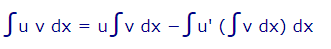• u is the function u(x)
• v is the function v(x)

As a diagram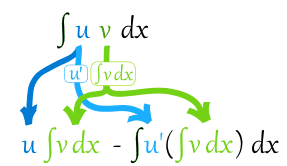Let's get straight into an example, and talk about it after:

### Example: What is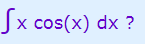?

OK, we have x multiplied by cos(x), so integration by parts is a good choice.

First choose which functions for u and v:

• u = x
• v = cos(x)

So now it is in the format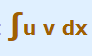we can proceed:

Differentiate u: u' = x' = 1

Integrate v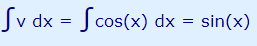(see Integration Rules)

Now we can put it togethe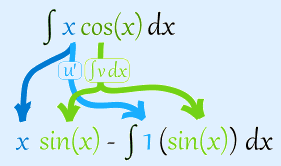Simplify and solve:

x sin(x) − ∫sin(x) dx

x sin(x) + cos(x) + C

So we followed these steps:

• Choose u and v
• Differentiate u: u'
• Integrate v: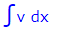• Put u, u' and ∫v dx into:u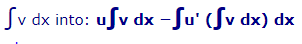• Simplify and solve

In English, to help you remember,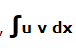becomes:

(u integral v) minus integral of (derivative u, integral v)

Let's try some more examples:

### Example: What is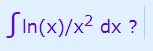First choose u and v:

• u = ln(x)
• v = 1/x2

Differentiate u: ln(x)' = 1/x

Integrate v: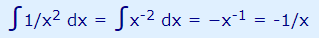(by the power rule)

Now put it together: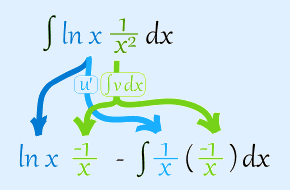Simplify:

− ln(x)/x −− (ln(x) + 1)/x + C

Example: What is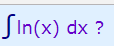But there is only one function! How do we choose u and v ?

Hey! We can just choose v as being "1":

• u = ln(x)
• v = 1

Differentiate u: ln(x)' = 1/x

Integrate v: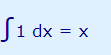Now put it together: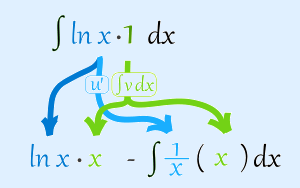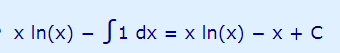Example: What is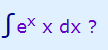Choose u and v:

• u = ex
• v = x

Differentiate u: (ex)' = ex

Integrate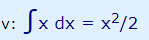Now put it together: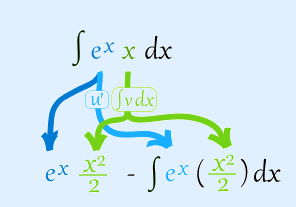Well, that was a spectacular disaster! It just got more complicated.

Maybe we could choose a different u and v?
Example: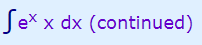Choose u and v differently:

• u = x
• v = ex

Differentiate u: (x)' = 1
Integrate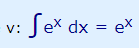Now put it together: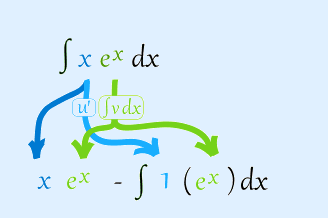Simplify:

x ex − ex + C

ex(x−1) + C

The moral of the story: Choose u and v carefully!

Choose a u that gets simpler when you differentiate it and a v that doesn't get any more complicated when you integrate it.

A helpful rule of thumb is I LATE. Choose u based on which of these comes first:

• I: Inverse trigonometric functions such as sin-1(x), cos-1(x), tan-1(x)
• L: Logarithmic functions such as ln(x), log(x)
• A: Algebraic functions such as x2, x3
• T: Trigonometric functions such as sin(x), cos(x), tan (x)
• E: Exponential functions such as ex, 3x

And here is one last (and tricky) example:
Example: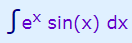• u = sin(x)
• v = ex

Differentiate u: sin(x)' = cos(x)
Integrate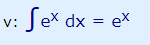Now put it together: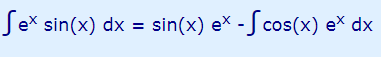Simplify: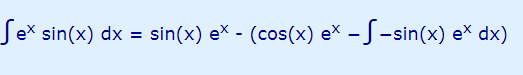Now we have the same integral on both sides (except one is subtracted) ...

... so bring the right hand one over to the left and we get: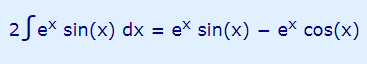Simplify: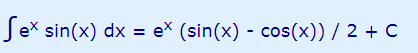## Footnote: Where Did "Integration by Parts" Come From?

It is based on the Product Rule for Derivatives:

(uv)' = uv' + u'v

Integrate both sides and rearrange: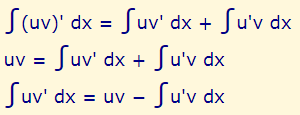Some people prefer that last form, but I like to integrate v' so the left side is simple: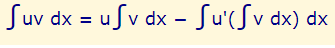Offer running on EduRev: Apply code STAYHOME200 to get INR 200 off on our premium plan EduRev Infinity!

122 videos|142 docs

,

,

,

,

,

,

,

,

,

,

,

,

,

,

,

,

,

,

,

,

,

,

,

,

;